About True and Vensim software In 2008, we found and downloaded the VensimPLE sofware. Without reading its manual, we analyzed its outputs to understand its principle. We discovered then that True software was based on the classical stock-and-flow principle, just as Vensim and Stella, with some differences of course. (Vensim (VensimPLE) is a software from Ventana Systems, Inc - Copyright © 1988-2011 Ventana Systems, Inc) Out of these, the most important are the following : Related to the Stock-and-Flow principle : Stocks : In Vensim, stocks change all together at the same time, only once by time unit, right before moving to the next time unit. In TRUE, stocks can be changed several times between two consecutive units of time, exactly when it is needed. Flows : In Vensim, the calculation order of the flows is automatic, strictly depending on the model building. There is one single clock for all flows. In TRUE, the calculation order of the flows is precisely determined by the user, according to the temporal parameters of each action of each flow, so that one could manage different clocks in the model. The principle used by Vensim permits/allows to easily calculate/compute a model in the continuous mode. For this, the values which change the stocks are divided according to the time step, and the number of time units is multiplied. If want it, TRUE can operate as Vensim. However, if the model contains several variations of the stocks between two consecutive time units, the modeling within the continuous mode cannot be automatic. Related to the causal loops : In Vensim, the causality links are displayed in advance, they are static. In TRUE, there are dynamic causality links displayed depending on the selected time unit and the values of the causal elements. These links accurately reflect the state of the model at a given time, after all the calculations have been done. Related to the model outputs in 2D, 3D and 4D : In TRUE, it is possible to select one unit of time and then to observe and analyze its corresponding values for all the stocks and flows of the model. So, it is possible to directly see in the model the step-by-step evolution of different variables, and therefore learn everything one cannot see in some database listings. In TRUE, it is also possible to create 3D scenes and then to animate them according to the variables of the model. These 3D scenes are then turned into 4D scenes, as movies. The 4D scenes allow the quick check of the behavior of any part of the model. They also enable the people which are not accustomed with the diagrams to better understand the models. Try to explain the behavior of this plane without 3D animation :Importing a Vensim (VensimPLE) modelImporting Vensim model Houses complete model ( see Vensim help chapter 11) Click on the image to display in Youtube Time system In Vensim : The time system seems to be a physical time system. The time system starts at Time = 0. Time is the only one unit of time. Example : One unit of time = one month Where : Time = 0 = January. In True : The time system is a calendar time system. The time system starts at Time = 1. Time = 0 is considered as the past. There is two units of time : Time and Cycle. Example : One unit of time = one month. One cycle = one year of 12 months. Where : Cycle 1 = 2012, Time = 1 = January 2012. Where : Time = 0 = end of december 2011. Stock equation In Vensim : A stock equation calculates the value that updates the stock. Example of stock equation : Houses In Construction = building-completing The stock is updated at the beginning of the unit of time. In continuous time, the value that updates the stock is automaticaly multiplied by the TIME STEP. In True : There is no stock equation. The value of the flow that links two stocks updates theses stocks. One stock is called the source stock, the other stock is called the target stock. The value of the flow is subtracted from the source stock and added to the target stock. The stocks are updated in real time, following each temporal parameters Chronology of the actions contained in the flow. In continuous time, the value of the flow that updates the stocks should be divided by the TimeStepI ( inverse of Vensim TIME STEP), In continuous time, if you enable the 'Rate' parameter of one action, the stocks will be updated as Vensim, at the end of the unit of time, by the value divided by TimeStepI. Stock initial value In Vensim : An equation calculates the initial value of the stock. Example of equation for initial value : Houses In Construction = building * time to build houses The initial value is calculated at the beginning of the first unit of time 0. In True : There is no equation that calculates the initial value of the stock. The initial value is a constant and can't be calculated. It is a previous data, the model can't change the past. The initial value is initialized for the unit of time 0. The unit of time 0 is the previous state of the model, before the first unit of time 1. When importing a Vensim model, the equation of the initial value of the stock is calculated and it is allocated to the stock as a constant value. Adding balancing stock In Vensim :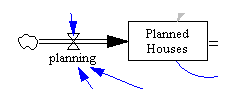A flow can 'indirectly update' a stock without updating another stock, because the return value of the stock equation updates the stock. Models in Vensim are not automaticaly balanced and they can be unbalanced. In True :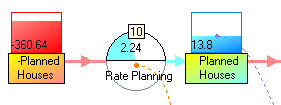A flow updates always two stocks together. With this principle, the sum o f all the stocks of the model is a constant. Models in True are automaticaly balanced and can't be unbalanced. When importing a Vensim model in True, balancing stocks are automaticaly added if necessary. Flow with action of type rate In Vensim :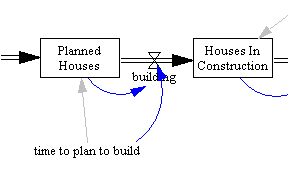The valve building doesn't update directly the stocks. The value that updates the stock is automaticaly multiplied by the TIME STEP. The stocks are updated at the beginning of the unit of time. The order of calculation of the variate and auxiliary, etc., is automatic. In True : To work as Vensim, first solution : - enable the 'Rate' parameter of the action of the flow Building.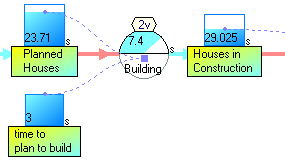To work as Vensim, second solution : - because the flow Building updates in real time the stocks, a second flow is added : - the equation of the new flow Rate Building is : y = Building / TimeStepI. - the chronology of the new flow is set to the highter chronology of the model. - the stocks will be updated at the end of the unit of time. The order of calculation of flow variables is not automatic, it is defined by the temporal parameters Chronology.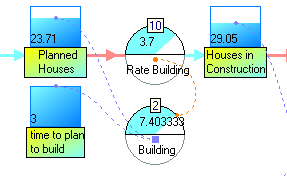Adding flow In Vensim :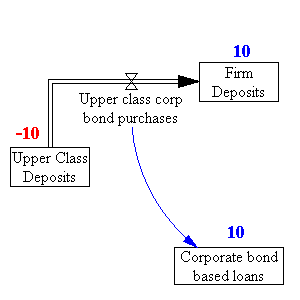Tree stocks are updated by one flow rate : Stock Corporate bond based loans = Upper class corp bond purchases Stock Firm Deposits = Upper class corp bond purchases Stock Upper Class Deposits = -Upper class corp bond purchases In Vensim it is possible to write unbalanced models. Example of results with following values : Final time = 10, TIME STEP = 1, Upper class corp bond purchases = 1, all stocks = 0 :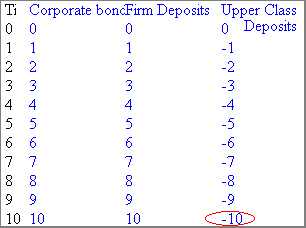In True :Because a flow can update only two stocks : the flow Upper class corp bond purchases is automaticaly duplicated, by adding the flow Upper class corp bond purchases1. In True it is not possible to update a stock without preserving equilibrium by updating another stock. In True the sum of all the stocks is constant. In True it is not possible to write unbalanced models. Example of results with following values : Final time = 10, TimeStepI = 1, Upper class corp bond purchases = 1, all stocks = 0 :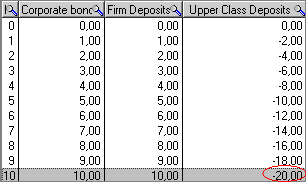Function equivalences